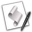## Number to Text Manipulations

Scripts often convert numeric data to text data in order to display information to the user. Most of the time this conversion can be accomplished simply by using the coercion handler as, like in the following example:

12 as string
--> Returns: "12"

However, some numeric values are displayed in scientific notation. For example, 1234000000 is displayed by a script as 1.234E+9

When this value is coerced to text it becomes: "1.234E+9"

The following sub-routine will convert any number to a string of numeric characters. This sub-routine is used by the other sub-routines on this page which also manipulate numeric data.

### Number to String

An easy-to-use sub-routine, just pass the number to convert to text as the passed parameter value in the sub-routine call:

number_to_string(8.72124243234E+11)
--> returns: "872124243234"

Here's the sub-routine:A sub-routine that converts numeric values to strings:

on number_to_string(this_number)
set this_number to this_number as string
if this_number contains "E+" then
set x to the offset of "." in this_number
set y to the offset of "+" in this_number
set z to the offset of "E" in this_number
set the decimal_adjust to characters (y - (length of this_number)) thru ¬
-1 of this_number as string as number
if x is not 0 then
set the first_part to characters 1 thru (x - 1) of this_number as string
else
set the first_part to ""
end if
set the second_part to characters (x + 1) thru (z - 1) of this_number as string
set the converted_number to the first_part
repeat with i from 1 to the decimal_adjust
try
set the converted_number to ¬
the converted_number & character i of the second_part
on error
set the converted_number to the converted_number & "0"
end try
end repeat
return the converted_number
else
return this_number
end if
end number_to_string

### Comma Delimit a Number

This sub-routine will comma delimit a numeric value and convert it to text.

comma_delimit(872124243234)
--> returns: "872,124,243,234"

NOTE: this sub-routine uses the number_to_sring() sub-routine shown above. Be sure to add it to your script as well.

Here's the sub-routine:A sub-routine that delimits numbers with commas:

on comma_delimit(this_number)
set this_number to this_number as string
if this_number contains "E" then set this_number to number_to_text(this_number)
set the num_length to the length of this_number
set the this_number to (the reverse of every character of this_number) as string
set the new_num to ""
repeat with i from 1 to the num_length
if i is the num_length or (i mod 3) is not 0 then
set the new_num to (character i of this_number & the new_num) as string
else
set the new_num to ("," & character i of this_number & the new_num) as string
end if
end repeat
return the new_num
end comma_delimit

### Round and Truncate

This sub-routine will round and truncate a numeric value and convert it to text. To use, pass the numeric value and the number of desired decimal places as the passed parameters.

round_truncate(1.04575, 3)
--> returns: "1.046"

NOTE: this sub-routine uses the number_to_sring() sub-routine shown above. Be sure to add it to your script as well.

Here's the sub-routine:A sub-routine that will round and truncate a passed number:

on round_truncate(this_number, decimal_places)
if decimal_places is 0 then
set this_number to this_number + 0.5
return number_to_text(this_number div 1)
end if

set the rounding_value to "5"
repeat decimal_places times
set the rounding_value to "0" & the rounding_value
end repeat
set the rounding_value to ("." & the rounding_value) as number

set this_number to this_number + rounding_value

set the mod_value to "1"
repeat decimal_places - 1 times
set the mod_value to "0" & the mod_value
end repeat
set the mod_value to ("." & the mod_value) as number

set second_part to (this_number mod 1) div the mod_value
if the length of (the second_part as text) is less than the decimal_places then
repeat decimal_places - (the length of (the second_part as text)) times
set second_part to ("0" & second_part) as string
end repeat
end if

set first_part to this_number div 1
set first_part to number_to_text(first_part)
set this_number to (first_part & "." & second_part)

return this_number
end round_truncate

The following sub-routine will add "st", "nd", "rd", or "th" to the end of a number.

--> returns: "24th"

Here's the sub-routine:A sub-routine for adding a descriptive suffix to the end of a numeric string:

set this_num to this_num as string
if this_num ends with "11" or this_num ends with "12" or this_num ends with "13" then
set the list_index to 1
else
set the list_index to (this_num mod 10) + 1
end if
set the num_suffix to item list_index of {"th", "st", "nd", "rd", "th", "th", "th", "th", "th", "th"}
return (this_num & num_suffix)

Here's an example script using the sub-routine:A script demonstrating the use of the sub-routine:

set this_list to {"Sal", "Carl", "Bob", "Sue", "Wanda"}
repeat with i from 1 to the count of this_list
display dialog "The " & add_numeric_suffix(i) & " person in the list is " & (item i of this_list) & "."
end repeat

The following sub-routine will place leading zeros (0001, 023, etc.) before a number.

For example, if the maximum number of leading zeros is set to 2, then the results will range from 001 to 999. If the maximum number of leading zeros is 3, then the results will range from 0001 to 9999, and so on.A sub-routine for adding leading zeros to numbers: